The Australian Journal of Mathematical Analysis and Applications

 Home News Editors Volumes RGMIA Subscriptions Authors Contact

ISSN 1449-5910

You searched for zhang
Total of 112 results found in site

13: Paper Source PDF document

Paper's Title:

Oscillations of First Order Linear Delay Difference Equations

Author(s):

G. E. Chatzarakis and I. P. Stavroulakis

Department of Mathematics, University of Ioannina,
451 10, Greece
ipstav@cc.uoi.gr

Abstract:

Consider the first order linear delay difference equation of the form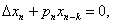Ā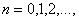Āwhere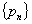Āis a sequence of nonnegative real numbers, k is a positive integer and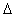Ādenotes the forward difference operator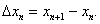ĀNew oscillation criteria are established when the well-known oscillation conditions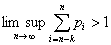Āand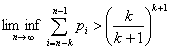Āare not satisfied. The results obtained essentially improve known results in the literature.

13: Paper Source PDF document

Paper's Title:

Existence Results for Perturbed Fractional Differential Inclusions

Author(s):

Y.-K. Chang

Department of Mathematics,
Lanzhou Jiaotong University, Lanzhou, Gansu 730070, People's
Republic of China
lzchangyk@163.com

Abstract:

This paper is mainly concerned with the following fractional differential inclusions with boundary condition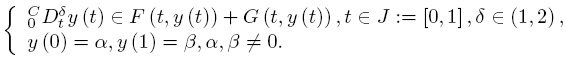A sufficient condition is established for the existence of solutions of the above problem by using a fixed point theorem for multivalued maps due to Dhage. Our result is proved under the mixed generalized Lipschitz and Carathķodory conditions.

5: Paper Source PDF document

Paper's Title:

A Geometric Generalization of Busemann-Petty Problem

Author(s):

Liu Rong and Yuan Jun

Shanghai Zhangjiang Group Junior Middle School,
China

Abstract:

The norm defined by Busemann's inequality establishes a class of star body - intersection body. This class of star body plays a key role in the solution of Busemann-Petty problem. In 2003, Giannapoulos  defined a norm for a new class of half-section. Based on this norm, we give a geometric generalization of Busemann-Petty problem, and get its answer as a result

5: Paper Source PDF document

Paper's Title:

On Subspace-Supercyclic Operators

Author(s):

Mansooreh Moosapoor

Assistant Professor,
Department of Mathematics,
Farhangian University, Tehran,
Iran.
E-mail: mosapor110@gmail.com m.mosapour@cfu.ac.ir

Abstract:

In this paper, we prove that supercyclic operators are subspace-supercyclic and by this we give a positive answer to a question posed in ( L. Zhang, Z. H. Zhou, Notes about subspace-supercyclic operators, Ann. Funct. Anal., 6 (2015), pp. 60--68). We give examples of subspace-supercyclic operators that are not subspace-hypercyclic. We state that if T is an invertible supercyclic operator then Tn and T-n is subspace-supercyclic for any positive integer n. We give two subspace-supercyclicity criteria. Surprisingly, we show that subspace-supercyclic operators exist on finite-dimensional spaces.

5: Paper Source PDF document

Paper's Title:

Hermite-Hadamard Type Inequalities for MN-Convex Functions

Author(s):

Sever S. Dragomir1,2

1Mathematics, College of Engineering & Science
Victoria University, PO Box 14428
Melbourne City, MC 8001,
Australia
E-mail: sever.dragomir@vu.edu.au

2DST-NRF Centre of Excellence in the Mathematical and Statistical Sciences,
School of Computer Science & Applied Mathematics,
University of the Witwatersrand,
Private Bag 3, Johannesburg 2050,
South Africa
URL: https://rgmia.org/dragomir

Abstract:

The present work endeavours to briefly present some of the fundamental results connected to the Hermite-Hadamard inequality for special classes of convex functions such as AG, AH, GA, GG, GH, HA, HG and HH -convex functions in which the author have been involved during the last five years. For simplicity, we call these classes of functions such as MN-convex functions, where M and N stand for any of the Arithmetic (A), Geometric (G) or Harmonic (H) weighted means of positive real numbers. The survey is intended for use by both researchers in various fields of Approximation Theory and Mathematical Inequalities, domains which have grown exponentially in the last decade, as well as by postgraduate students and scientists applying inequalities in their specific areas.

4: Paper Source PDF document

Paper's Title:

Existence of Positive Solutions for Nonlinear Fractional Differential Equations with Multi-point Boundary Conditions

Author(s):

Khenchela University, Department of Mathematics,
Khenchela, 40000,
Algeria.

Abstract:

This paper is devoted to the existence results of positive solutions for a nonlinear fractional differential equations with multi-point boundary conditions. By means of the Schauder fixed point theorem, some results on the existence are obtained.

4: Paper Source PDF document

Paper's Title:

Cubic Alternating Harmonic Number Sums

Author(s):

Anthony Sofo

Victoria University,
College of Engineering and Science,
Melbourne City,
Australia.
E-mail: Anthony.Sofo@vu.edu.au

Abstract:

We develop new closed form representations of sums of cubic alternating harmonic numbers and reciprocal binomial coefficients. We also identify a new integral representation for the ζ (4)  constant.

4: Paper Source PDF document

Paper's Title:

Fractional exp(-φ(ξ))- Expansion Method and its Application to Space--Time Nonlinear Fractional Equations

Author(s):

A. A. Moussa and L. A. Alhakim

Department of Management Information System and Production Management,
College of Business and Economics, Qassim University,
P.O. BOX 6666, Buraidah: 51452,
Saudi Arabia.
E-mail: Alaamath81@gmail.com

Department of Management Information System and Production Management,
College of Business and Economics, Qassim University,
P.O. BOX 6666, Buraidah: 51452,
Saudi Arabia.
E-mail: Lama2736@gmail.com

Abstract:

In this paper, we mainly suggest a new method that depends on the fractional derivative proposed by Katugampola for solving nonlinear fractional partial differential equations. Using this method, we obtained numerous useful and surprising solutions for the space--time fractional nonlinear Whitham--Broer--Kaup equations and space--time fractional generalized nonlinear Hirota--Satsuma coupled KdV equations. The solutions obtained varied between hyperbolic, trigonometric, and rational functions, and we hope those interested in the real-life applications of the previous two equations will find this approach useful.

3: Paper Source PDF document

Paper's Title:

A Simple New Proof of Fan-Taussky-Todd Inequalities

Author(s):

Zhi-Hua Zhang and Zhen-Gang Xiao

Zixing Educational Research Section,
Chenzhou City, Hunan 423400, P. R. China.
Zhi-hua Zhang
Url: http://www.hnzxslzx.com/zzhweb/

Department Of Mathematics, Hunan Institute Of Science And Technology,
Yueyang City, Hunan 423400, P. R. China.
Zhen-gang Xiao

Abstract:

In this paper we present simple new proofs of the inequalities: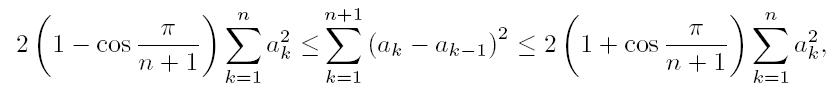which holds for all real numbers a0 = 0, a1, Ę Ę Ę , an, an+1 = 0 and the coefficients 2(1 - cos(π/(n + 1))) and 2(1 + cos(π/(n + 1))) are the best possible; and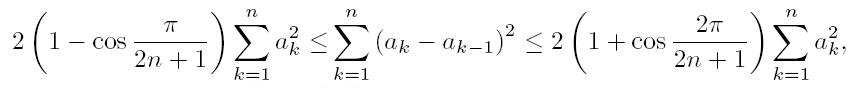which holds for all real numbers a0 = 0, a1, Ę Ę Ę , an and the coefficients 2(1-cos(π/(2n + 1))) and 2(1 + cos(π/(2n + 1))) are the best possible.

3: Paper Source PDF document

Paper's Title:

On Pseudo Almost Periodic Solutions to Some Neutral Functional-Differential Equations

Author(s):

Toka Diagana and Eduardo Hernßndez

Department of Mathematics, Howard University
2441 6th Street NW, Washington DC 20059,
USA.
tdiagana@howard.edu

Departamento de Matemßtica, I.C.M.C. Universidade de SŃo Paulo,
Caixa Postal 668, 13560-970, SŃo Carlos SP,
Brazil.
lalohm@icmc.sc.usp.br

Abstract:

This paper discusses the existence and uniqueness of pseudo almost periodic solutions to a class of partial neutral functional-differential equations. Under some suitable assumptions, existence and uniqueness results are obtained. An example is given to illustrate abstract results.

3: Paper Source PDF document

Paper's Title:

On Oscillation of Second-Order Delay Dynamic Equations on Time Scales

Author(s):

S. H. Saker

Department of Mathematics, Faculty of Science,
Mansoura University, Mansoura, 35516,
Egypt.
shsaker@mans.edu.eg

Abstract:

Some new oscillation criteria for second-order linear delay dynamic equation on a time scale T are established. Our results improve the recent results for delay dynamic equations and in the special case when T=R, the results include the oscillation results established by Hille [1948, Trans. Amer. Math. Soc. 64 (1948), 234-252] and Erbe [Canad. Math. Bull. 16 (1973), 49-56.] for differential equations. When T=Z the results include and improve some oscillation criteria for difference equations. When T=hZ, h>0, T=qN and T=N2, i.e., for generalized second order delay difference equations our results are essentially new and can be applied on different types of time scales. An example is considered to illustrate the main results.

3: Paper Source PDF document

Paper's Title:

On Some Remarkable Product of Theta-function

Author(s):

M. S. Mahadeva Naika, M. C. Maheshkumar and K. Sushan Bairy

Department of Mathematics,
Bangalore University, Central College Campus,
Bangalore-560 001,
INDIA
msmnaika@rediffmail.com
softmahe@rediffmail.com
ksbairy@gmail.com

Abstract:

On pages 338 and 339 in his first notebook, Ramanujan records eighteen values for a certain product of theta-function. All these have been proved by B. C. Berndt, H. H. Chan and L-C. Zhang . Recently M. S. Mahadeva Naika and B. N. Dharmendra [7, 8] and Mahadeva Naika and M. C. Maheshkumar  have obtained general theorems to establish explicit evaluations of Ramanujan's remarkable product of theta-function. Following Ramanujan we define a new function bM,N as defined in (1.5). The main purpose of this paper is to establish some new general theorems for explicit evaluations of product of theta-function.

3: Paper Source PDF document

Paper's Title:

Positive Periodic Solutions for Second-Order Differential Equations with Generalized Neutral Operator

Author(s):

Wing-Sum Cheung, Jingli Ren and Weiwei Han

Department of Mathematics,
The University of Hong Kong
Hong Kong

Department of Mathematics, Zhengzhou University
Zhengzhou 450001,
P.R. China

wscheung@hkucc.hku.hk
renjl@zzu.edu.cn

Abstract:

By some analysis of the neutral operator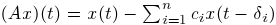and an application of the fixed-point index theorem, we obtain sufficient conditions for the existence, multiplicity and nonexistence of periodic solutions to a second-order differential equation with the prescribed neutral operator, which improve and extend some recent results of Lu-Ge, Wu-Wang, and Zhang. An example is given to illustrate our results. Moreover, the analysis of the generalized neutral operator will be helpful for other types of differential equations.

3: Paper Source PDF document

Paper's Title:

New Implicit Kirk-Type Schemes for General Class of Quasi-Contractive Operators in Generalized Convex Metric Spaces

Author(s):

K. Rauf, O. T. Wahab and A. Ali

Department of Mathematics,
University of Ilorin, Ilorin,
Nigeria.
E-mail: krauf@unilorin.edu.ng

Department of Statistics and Mathematical Sciences,
Kwara State University, Malete,
Nigeria.

Department of Mathematics,
Mirpur University of Science and Technology, Mirpur,
Pakistan.

Abstract:

In this paper, we introduce some new implicit Kirk-type iterative schemes in generalized convex metric spaces in order to approximate fixed points for general class of quasi-contractive type operators. The strong convergence, T-stability, equivalency, data dependence and convergence rate of these results were explored. The iterative schemes are faster and better, in term of speed of convergence, than their corresponding results in the literature. These results also improve and generalize several existing iterative schemes in the literature and they provide analogues of the corresponding results of other spaces, namely: normed spaces, CAT(0) spaces and so on.

2: Paper Source PDF document

Paper's Title:

Positive Periodic Time-Scale Solutions for Functional Dynamic Equations

Author(s):

Douglas R. Anderson and Joan Hoffacker

Department of Mathematics and Computer Science
Concordia College
andersod@cord.edu
URL:
http://www.cord.edu/faculty/andersod/

Department of Mathematical Sciences
Clemson University
Clemson, SC 29634 USA
johoff@clemson.edu
URL:
http://www.math.clemson.edu/facstaff/johoff.htm

Abstract:

Using Krasnoselskii's fixed point theorem, we establish the existence of positive periodic solutions to two pairs of related nonautonomous functional delta dynamic equations on periodic time scales, and then extend the discussion to higher-dimensional equations. Two pairs of corresponding nabla equations are also provided in an analogous manner.

2: Paper Source PDF document

Paper's Title:

Generalized Quasilinearization Method for the Forced D³ffing Equation

Author(s):

Ramzi S. N. Alsaedi

Department of Mathematics, King Abdul Aziz University,
Jeddah P.O. Box 80203,
Saudi Arabia.
ramzialsaedi@yahoo.co.uk

Abstract:

A generalized quasilinearization method for the periodic problem related to the forced D\"{u}ffing equation is developed and a sequence of approximate solutions converging monotonically and quadratically to the solution of the given problem is presented.

2: Paper Source PDF document

Paper's Title:

Asymptotic Distribution of Products of Weighted Sums of Dependent Random Variables

Author(s):

Y. Miao and J. F. Li

College of Mathematics and Information Science,
Henan Normal University
Henan, China
yumiao728@yahoo.com.cn

College of Mathematics and Information Science,
Henan Normal University, 453007
Henan, China.
junfen_li@yahoo.com.cn

Abstract:

In this paper we establish the asymptotic distribution of products of weighted sums of dependent positive random variable, which extends the results of Rempała and Wesołowski (2002).

2: Paper Source PDF document

Paper's Title:

Nontrivial Solutions of Singular Superlinear Three-point Boundary Value Problems at Resonance

Author(s):

Feng Wang, Fang Zhang

School of Mathematics and Physics,
Changzou University,
Changzhou, 213164,
China.
fengwang188@163.com

Abstract:

The singular superlinear second order three-point boundary value problems at resonance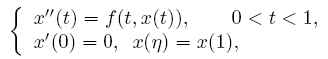are considered under some conditions concerning the first eigenvalues corresponding to the relevant linear operators, where n ∈ (0,1) is a constant, f is allowed to be singular at both t=0 and t=1. The existence results of nontrivial solutions are given by means of the topological degree theory.

2: Paper Source PDF document

Paper's Title:

On A Conjecture of A Logarithmically Completely Monotonic Function

Author(s):

Valmir Krasniqi, Armend Sh. Shabani

Department of Mathematics,
University of Prishtina,
Republic of Kosova

E-mail: vali.99@hotmail.com
armend_shabani@hotmail.com

Abstract:

In this short note we prove a conjecture, related to a logarithmically completely monotonic function, presented in . Then, we extend by proving a more generalized theorem. At the end we pose an open problem on a logarithmically completely monotonic function involving q-Digamma function.

2: Paper Source PDF document

Paper's Title:

Credibility Based Fuzzy Entropy Measure

Author(s):

G. Yari, M. Rahimi, B. Moomivand and P. Kumar

Department of Mathematics,
Iran University of Science and Technology,
Tehran,
Iran.
E-mail: Yari@iust.ac.ir
E-mail: Mt_Rahimi@iust.ac.ir
URL: http://www.iust.ac.ir/find.php?item=30.11101.20484.en
URL: http://webpages.iust.ac.ir/mt_rahimi/en.html

Qarzol-hasaneh
Mehr Iran Bank, Tehran,
Iran.
E-mail: B.moomivand@qmb.ir

Department of Mathematics and Statistics,
University of Northern British Columbia,
Prince George, BC,
E-mail: Pranesh.Kumar@unbc.ca

Abstract:

Fuzzy entropy is the entropy of a fuzzy variable, loosely representing the information of uncertainty. This paper, first examines both previous membership and credibility based entropy measures in fuzzy environment, and then suggests an extended credibility based measure which satisfies mostly in Du Luca and Termini axioms. Furthermore, using credibility and the proposed measure, the relative entropy is defined to measure uncertainty between fuzzy numbers. Finally we provide some properties of this Credibility based fuzzy entropy measure and to clarify, give some examples.

2: Paper Source PDF document

Paper's Title:

New Refinements of H÷lder's Inequality

Author(s):

Xiu-Fen Ma

College of Mathematical and Computer,
No.9 of Xuefu Road, Hechuan District 401520,
Chongqing City,
The People's Republic of China.
E-mail: maxiufen86@163.com

Abstract:

In this paper, we define two mappings, investigate their properties, obtain some new refinements of H÷lder's inequality.

2: Paper Source PDF document

Paper's Title:

Mapped Chebyshev Spectral Methods for Solving Second Kind Integral Equations on the Real Line

Author(s):

Ahmed Guechi and Azedine Rahmoune

Department of Mathematics, University of Bordj Bou Arrķridj,
El Anasser, 34030, BBA,
Algeria.
E-mail: a.guechi2017@gmail.com
E-mail: a.rahmoune@univ-bba.dz

Abstract:

In this paper we investigate the utility of mappings to solve numerically an important class of integral equations on the real line. The main idea is to map the infinite interval to a finite one and use Chebyshev spectral-collocation method to solve the mapped integral equation in the finite interval. Numerical examples are presented to illustrate the accuracy of the method.

2: Paper Source PDF document

Paper's Title:

Convergence Speed of Some Random Implicit-Kirk-type Iterations for Contractive-type Random Operators

Author(s):

H. Akewe, K.S. Eke

Department of Mathematics,
Covenant University,
Canaanland, KM 10, Idiroko Road, P. M. B. 1023, Ota, Ogun State,
Nigeria.
E-mail: hudson.akewe@covenantuniversity.edu.ng, kanayo.eke@covenantuniversity.edu.ng

Abstract:

The main aim of this paper is to introduce a stochastic version of multistep type iterative scheme called a modified random implicit-Kirk multistep iterative scheme and prove strong convergence and stability results for a class of generalized contractive-type random operators. The rate of convergence of the random iterative schemes are also examined through an example. The results show that our new random implicit kirk multistep scheme perform better than other implicit iterative schemes in terms of convergence and thus have good potentials for further applications in equilibrium problems in computer science, physics and economics.

2: Paper Source PDF document

Paper's Title:

Global Analysis on Riemannian Manifolds

Author(s):

Louis Omenyi and Michael Uchenna

Department of Mathematics, Computer Science, Statistics and Informatics,
Alex Ekwueme Federal University, Ndufu-Alike,
Nigeria.
E-mail: omenyi.louis@funai.edu.ng, michael.uchenna@funai.edu.ng
URL: http://www.funai.edu.ng

Abstract:

In this paper, an exposition of the central concept of global analysis on a Riemannan manifold is given. We extend the theory of smooth vector fields from open subsets of Euclidean space to Riemannan manifolds. Specifically, we prove that a Riemannian manifold admits a unique solution for a system of ordinary differential equations generated by the flow of smooth tangent vectors. The idea of partial differential equations on Riemannian manifold is highlighted on the unit sphere.

1: Paper Source PDF document

Paper's Title:

Multivalued Equilibrium Problems with Trifunction

Author(s):

Etisalat College of Engineering, P.O. Box 980, Sharjah, United Arab Emirates
noor@ece.ac.ae

Abstract:

In this paper, we use the auxiliary principle technique to suggest some new classes of iterative algorithms for solving multivalued equilibrium problems with trifunction. The convergence of the proposed methods either requires partially relaxed strongly monotonicity or pseudomonotonicity. As special cases, we obtain a number of known and new results for solving various classes of equilibrium and variational inequality problems. Since multivalued equilibrium problems with trifunction include equilibrium, variational inequality and complementarity problems as specials cases, our results continue to hold for these problems.

1: Paper Source PDF document

Paper's Title:

Fekete-Szeg÷ Inequality for Certain Class of Analytic Functions

Author(s):

V. Ravichandran, Maslina Darus, M. Hussain Khan, and  K. G. Subramanian

School of Mathematical Sciences, Universiti Sains Malaysia,
11800 Usm, Penang, Malaysia

School of Mathematical Sciences, Faculty of Sciences and Technology,
Ukm, Banki 43600, Malaysia

Department of Mathematics, Islamiah College,
V

Department of Mathematics, Madras Christian College, Tambaram,
Chennai- 600 059, India

Abstract:

In this present investigation, the authors obtain Fekete-Szeg÷ inequality for a certain class of analytic functions f(z) for which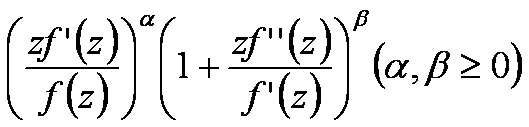lies in a region starlike with respect to 1 and symmetric with respect to the real axis. Also certain application of our main result for a class of functions defined by Hadamard product (convolution) is given. As a special case of our result we obtain Fekete-Szeg÷ inequality for a class of functions defined through fractional derivatives. Also we obtain Fekete-Szeg÷ inequality for the inverse functions.

1: Paper Source PDF document

Paper's Title:

On the Fekete-Szegő Inequality for Some Subclasses of Analytic Functions

Author(s):

T.N. Shanmugam and A. Singaravelu

Department of Mathematics,
College of Engineering,
Anna University, Chennai-600 025,
shan@annauniv.edu

Department of Mathematics,
Valliammai Engineering College,
Chennai-603 203,
sivasaisastha@rediffmail.com

Abstract:

In this present investigation, the authors obtainFekete-Szegő's inequality for certain normalized analytic functions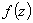defined on the open unit disk for which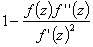lie in a region starlike with respect to 1 and symmetric with respect to the real axis. Also certain applications of the main result for a class of functions defined by convolution are given. As a special case of this result, Fekete-Szegő's inequality for a class of functions defined through fractional derivatives is also obtained.

1: Paper Source PDF document

Paper's Title:

A New Step Size Rule in Noor's Method for Solving General Variational Inequalities

Author(s):

Abdellah Bnouhachem

School of Management Science and Engineering, Nanjing University,
Nanjing, 210093
P.R. China.
babedallah@yahoo.com

Abstract:

In this paper, we propose a new step size rule in Noor's method for solving general variational inequalities. Under suitable conditions, we prove that the new method is globally convergent. Preliminary numerical experiments are included to illustrate the advantage and efficiency of the proposed method.

1: Paper Source PDF document

Paper's Title:

Local and Global Existence and Uniqueness Results for Second and Higher Order Impulsive Functional Differential Equations with Infinite Delay

Author(s):

Johnny Henderson and Abdelghani Ouahab

Department of Mathematics, Baylor University,
Waco, Texas 76798-7328
USA.
Johnny_Henderson@baylor.edu

Laboratoire de Mathķmatiques, Universitķ de Sidi Bel Abbķs
BP 89, 22000 Sidi Bel Abbķes,
Algķrie.
ouahab@univ-sba.dz

Abstract:

In this paper, we discuss the local and global existence and uniqueness results for second and higher order impulsive functional differential equations with infinite delay. We shall rely on a nonlinear alternative of Leray-Schauder. For the global existence and uniqueness we apply a recent Frigon and Granas nonlinear alternative of Leray-Schauder type in Frķchet spaces.

1: Paper Source PDF document

Paper's Title:

An Approximation of Jordan Decomposable Functions for a Lipschitz Function

Author(s):

Ibraheem Alolyan

Mathematics Department,
College of Science, King Saud University
Saudi Arabia
ialolyan05@yahoo.com

Abstract:

The well known Jordan decomposition theorem gives the useful characterization that any function of bounded variation can be written as the difference of two increasing functions. Functions which can be expressed in this way can be used to formulate an exclusion test for the recent Cellular Exclusion Algorithms for numerically computing all zero points or the global minima of functions in a given cellular domain [2,8,9]. In this paper we give an algorithm to approximate such increasing functions when only the values of the function of bounded variation can be computed. For this purpose, we are led to introduce the idea of ε-increasing functions. It is shown that for any Lipschitz continuous function, we can find two ε-increasing functions such that the Lipschitz function can be written as the difference of these functions.

1: Paper Source PDF document

Paper's Title:

On the Asymptotic Behavior of Solutions of Third Order Nonlinear Differential Equations

Author(s):

Ivan Mojsej and Alena Tartaľovß

Institute of Mathematics,
Faculty of Science, P. J. Ŗafßrik University,
Jesennß 5, 041 54 KoÜice,
Slovak Republic
ivan.mojsej@upjs.sk

Department of Applied Mathematics and Business Informatics,
Faculty of Economics, Technical University,
Nemcovej 32, 040 01 KoÜice,
Slovak Republic
alena.tartalova@tuke.sk

Abstract:

This paper is concerned with the asymptotic behavior of solutions of nonlinear differential equations of the third order with quasiderivatives. Mainly, we present the necessary and sufficient conditions for the existence of nonoscillatory solutions with specified asymptotic behavior as t tends to infinity. These conditions are presented as integral criteria and involve only the coefficients of investigated differential equations. In order to prove some of the results, we use a topological approach based on the Schauder fixed point theorem.

1: Paper Source PDF document

Paper's Title:

Equilibria and Periodic Solutions of Projected Dynamical Systems on Sets with Corners

Author(s):

Matthew D. Johnston and Monica-Gabriela Cojocaru

Department of Applied Mathematics, University of Waterloo,
mdjohnst@math.uwaterloo.ca

Department of Mathematics & Statistics, University of Guelph,
mcojocar@uoguelph.ca

Abstract:

Projected dynamical systems theory represents a bridge between the static worlds of variational inequalities and equilibrium problems, and the dynamic world of ordinary differential equations. A projected dynamical system (PDS) is given by the flow of a projected differential equation, an ordinary differential equation whose trajectories are restricted to a constraint set K. Projected differential equations are defined by discontinuous vector fields and so standard differential equations theory cannot apply. The formal study of PDS began in the 90's, although some results existed in the literature since the 70's. In this paper we present a novel result regarding existence of equilibria and periodic cycles of a finite dimensional PDS on constraint sets K, whose points satisfy a corner condition. The novelty is due to proving existence of boundary equilibria without using a variational inequality approach or monotonicity type conditions.

1: Paper Source PDF document

Paper's Title:

Fekete-Szeg÷ Problem for Univalent Functions with Respect to k-Symmetric Points

Author(s):

K. Al-Shaqsi and M. Darus

School of Mathematical Sciences, Faculty of Science and Technology,
University Kebangsaan Malaysia,
Bangi 43600 Selangor D. Ehsan,
Malaysia
ommath@hotmail.com
maslina@ukm.my

Abstract:

In the present investigation, sharp upper bounds of |a3- μa22| for functions f(z) = z + a2z2 + a2z3 + ... belonging to certain subclasses of starlike and convex functions with respect to k-symmetric points are obtained. Also certain applications of the main results for subclasses of functions defined by convolution with a normalized analytic function are given. In particular, Fekete- Szeg÷ inequalities for certain classes of functions defined through fractional derivatives are obtained.

1: Paper Source PDF document

Paper's Title:

On a Method of Proving the Hyers-Ulam Stability of Functional Equations on Restricted Domains

Author(s):

Janusz Brzdęk

Department of Mathematics
Pedagogical University Podchor
ąźych 2,
30-084 Krak
¾w,
Poland
jbrzdek@ap.krakow.pl

Abstract:

We show that generalizations of some (classical) results on the Hyers-Ulam stability of functional equations, in several variables, can be very easily derived from a simple result on stability of a functional equation in single variable

1: Paper Source PDF document

Paper's Title:

Applications of Relations and Relators in the Extensions of Stability Theorems for Homogeneous and Additive Functions

Author(s):

rpßd Szßz

Institute of Mathematics, University of Debrecen,
H-4010 Debrecen, Pf. 12,
Hungary
szaz@math.klte.hu

Abstract:

By working out an appropriate technique of relations and relators and extending the ideas of the direct methods of Z. Gajda and R. Ger, we prove some generalizations of the stability theorems of D. H. Hyers, T. Aoki, Th. M. Rassias and P. Găvruţă in terms of the existence and unicity of 2-homogeneous and additive approximate selections of generalized subadditive relations of semigroups to vector relator spaces. Thus, we obtain generalizations not only of the selection theorems of Z. Gajda and R. Ger, but also those of the present author.

1: Paper Source PDF document

Paper's Title:

Existence Results for Second Order Impulsive Functional Differential Equations with Infinite Delay

Author(s):

M. Lakrib, A. Oumansour and K. Yadi

Laboratoire de Mathķmatiques, Universitķ Djillali
Liabķes, B.P. 89 Sidi Bel AbbĶs 22000, Algķrie
mlakrib@univ-sba.dz
oumansour@univ-sba.dz

Laboratoire de Mathķmatiques, Universitķ Abou Bekr
Belkaid, B.P. 119 Tlemcen 13000, Algķrie

Abstract:

In this paper we study the existence of solutions for second order impulsive functional differential equations with infinite delay. To obtain our results, we apply fixed point methods.

1: Paper Source PDF document

Paper's Title:

Some New Nonlinear Integro-Differential Inequalities of Gronwall-Bellman-Pachpatte Type

Author(s):

A. ABDELDAIM

Department of Mathematics and Computer Sciences,
Faculty of Science,
Port Said University, Port Said,
EGYPT.

Department of Mathematics,
Faculty of Science and Humanities,
SAUDI ARABIA.

E-mail: ahassen@su.edu.sa
URL: http://faculty.ksu.edu.sa/DRABDELDAIM/Pages/Home.aspx

Abstract:

In this paper we establish some new nonlinear integro-differential inequalities of Gronwall-Bellman-Pachpatte type for function of one independent variable. The purpose of this paper is to extend certain results which proved by Pachpatte in [On some fundamental integrodifferential and integral inequalities, An. Sti. Univ. Al. I. Cuza, Iasi, Vol.23 (1977), 77-86]. The inequalities obtained here can be used in the theory of some new classes of nonlinear integro-differential equations. Some applications are also given to illustrate the usefulness of our results.

1: Paper Source PDF document

Paper's Title:

Stability of an Almost Surjective epsilon-Isometry in The Dual of Real Banach Spaces

Author(s):

Minanur Rohman, Ratno Bagus Edy Wibowo, Marjono

Department of Mathematics, Faculty of Mathematics and Natural Sciences,
Brawijaya University,
Jl. Veteran Malang 65145,
Indonesia.
E-mail: miminanira@gmail.com

Department of Mathematics, Faculty of Mathematics and Natural Sciences,
Brawijaya University,
Jl. Veteran Malang 65145,
Indonesia.
E-mail: rbagus@ub.ac.id

Department of Mathematics, Faculty of Mathematics and Natural Sciences,
Brawijaya University,
Jl. Veteran Malang 65145,
Indonesia.
E-mail: marjono@ub.ac.id

Abstract:

In this paper, we study the stability of epsilon-isometry in the dual of real Banach spaces. We prove that the almost surjective epsilon-isometry mapping is stable in dual of each spaces. The proof uses GŌteaux differentiability space (GDS), weak-star exposed points, norm-attaining operator, and some studies about epsilon-isometry that have been done before.

1: Paper Source PDF document

Paper's Title:

Wavelet Frames in Higher Dimensional Sobolev Spaces

Author(s):

Raj Kumar, Manish Chauhan, and Reena

Department of Mathematics,
Kirori Mal College, University of Delhi,
New Delhi-110007,
India.
E-mail: rajkmc@gmail.com

Department of Mathematics,
University of Delhi,
New Delhi-110007,
India
E-mail: manish17102021@gmail.com

Department of Mathematics,
Hans Raj College, University of Delhi,
New Delhi-110007,
India
E-mail: reena.bhagwat29@gmail.com

Abstract:

In this paper, we present sufficient condition for the sequence of vectors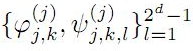to be a frame for Hs(Rd) are derived. Necessary and sufficient conditions for the sequence of vectorsto be tight wavelet frames in Hs(Rd) are obtained. Further, as an application an example of tight wavelet frames for Hs(R2) as bivariate box spline over 3-direction are given.

1: Paper Source PDF document

Paper's Title:

Author(s):

F. U. Ogbuisi

School of Mathematics, Statistics and Computer Science,
University of KwaZulu-Natal, Durban,
South Africa.

Department of Mathematics,
University of Nigeria, Nsukka,
Nigeria.
E-mail: ferdinard.ogbuisi@unn.edu.ng fudochukwu@yahoo.com

Abstract:

In this paper, we propose and analyze a type of subgradient extragradient algorithm for the approximation of a solution of variational inequality problem which is also a common fixed point of an infinite family of relatively nonexpansive mappings in 2-uniformly convex Banach spaces which are uniformly smooth. By using the generalized projection operator, we prove a strong convergence theorem which does not require the prior knowledge of the Lipschitz constant of cost operator. We further applied our result to constrained convex minimization problem, convex feasibility problem and infinite family of equilibrium problems. Our results improve and complement related results in 2-uniformly convex and uniformly smooth Banach spaces and Hilbert spaces.

1: Paper Source PDF document

Paper's Title:

Reduced Generalized Combination Synchronization Between Two n-Dimensional Integer-Order Hyperchaotic Systems and One m-Dimensional Fractional-Order Chaotic System

Author(s):

Smail Kaouache, Mohammed Salah Abdelouahab and Rabah Bououden

Laboratory of Mathematics and their interactions,
Abdelhafid Boussouf University Center, Mila.
Algeria
E-mail: smailkaouache@gmail.com, medsalah3@yahoo.fr, rabouden@yahoo.fr

Abstract:

This paper is devoted to investigate the problem of reduced generalized combination synchronization (RGCS) between two n-dimensional integer-order hyperchaotic drive systems and one m-dimensional fractional-order chaotic response system. According to the stability theorem of fractional-order linear system, an active mode controller is proposed to accomplish this end. Moreover, the proposed synchronization scheme is applied to synchronize three different chaotic systems, which are the Danca hyperchaotic system, the modified hyperchaotic Rossler system, and the fractional-order Rabinovich-Fabrikant chaotic system. Finally, numerical results are presented to fit our theoretical analysis.

1: Paper Source PDF document

Paper's Title:

Existence and Approximation of Traveling Wavefronts for the Diffusive Mackey-Glass Equation

Author(s):

C. Ramirez-Carrasco and J. Molina-Garay

Chile
E-mail: carloshrc1989@gmail.com
molina@imca.edu.pe

Abstract:

In this paper, we consider the diffusive Mackey-Glass model with discrete delay. This equation describes the dynamics of the blood cell production. We investigate the existence of traveling wavefronts solutions connecting the two steady states of the model. We develop an alternative proof of the existence of such solutions and we also demonstrate the existence of traveling wavefronts moving at minimum speed. The proposed approach is based on the use technique of upper-lower solutions. Finally, through an iterative procedure, we show numerical simulations that approximate the traveling wavefronts, thus confirming our theoretical results.

1: Paper Source PDF document

Paper's Title:

Coexisting Attractors and Bubbling Route to Chaos in Modified Coupled Duffing Oscillators

Author(s):

B. Deruni1, A. S. Hacinliyan1,2, E. Kandiran3, A. C. Keles2, S. Kaouache4, M.-S. Abdelouahab4, N.-E. Hamri4

1Department of Physics,
University of Yeditepe,
Turkey.

2Department of Information Systems and Technologies,
University of Yeditepe,
Turkey

3Department of Software Development,
University of Yeditepe,
Turkey.

4Laboratory of Mathematics and their interactions,
University Center of Abdelhafid Boussouf,
Mila 43000,
Algeria.

E-mail: berc890@gmail.com
ahacinliyan@yeditepe.edu.tr
engin.kandiran@yeditepe.edu.tr
cihan.keles@yeditepe.edu.tr
s.kaouache@centr-univ-mila.dz
medsalah3@yahoo.fr
n.hamri@centre-univ-mila.dz

Abstract:

In this article dynamical behavior of coupled Duffing oscillators is analyzed under a small modification. The oscillators have cubic damping instead of linear one. Although single duffing oscillator has complex dynamics, coupled duffing systems possess a much more complex structure. The dynamical behavior of the system is investigated both numerically and analytically. Numerical results indicate that the system has double scroll attractor with suitable parameter values. On the other hand, bifurcation diagrams illustrate rich behavior of the system, and it is seen that, system enters into chaos with different routes. Beside classical bifurcations, bubbling route to chaos is observed for suitable parameter settings. On the other hand, Multistability of the system is indicated with the coexisting attractors, such that under same parameter setting the system shows different periodic and chaotic attractors. Moreover, chaotic synchronization of coupled oscillators is illustrated in final section.

1: Paper Source PDF document

Paper's Title:

Riemann-Stieltjes Integrals and Some Ostrowski Type Inequalities

Author(s):

W. G. Alshanti

Department of General Studies,
Jubail University College,
KSA.
E-mail: shantiw@ucj.edu.sa

Abstract:

In this article, we investigate new integral inequalities of Ostrowski's type of various functional aspects. For mapping's second derivative, we assume two cases, namely, L1 and L spaces. Moreover, for first derivative, we investigate two different characteristics, namely, bounded variation and locally Lipchitz continuity. Applications to special means and composite quadrature rules are also carried out.

1: Paper Source PDF document

Paper's Title:

Coefficient Estimates Of Sakaguchi Kind Functions Using Lucas Polynomials

Author(s):

H. Priya and B. Srutha Keerthi

Department of Mathematics,
VIT Chennai Campus,
Chennai - 600 048,
India.
E-mail: priyaharikrishnan18@gmail.com

Department of Mathematics,
VIT Chennai Campus,
Chennai - 600 048,
India.
E-mail: i
sruthilaya06@yahoo.co.in

Abstract:

By means of (p,q) Lucas polynomials, we estimate coefficient bounds and Fekete-Szego inequalities for functions belonging to this class. Several corollaries and consequences of the main results are also obtained.

Search and serve lasted 0 second(s).

® 2004-2021 Austral Internet Publishing